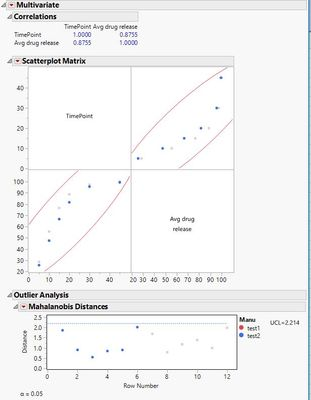Choose Language Hide Translation Bar
Highlighted

## Multivariate Statistical Distance (MSD)

Hi,

Is there a way to calculate the multivariate statistical distance (MSD) in JMP? This calculation will help understand the difference between dissoulutions.

Thank you,

Rami

10 REPLIES 10
Highlighted

## Re: Multivariate Statistical Distance (MSD)

There are many ways to calculate a distance. A distance measure is the basis for many multivariate methods. What method are you thinking of using?

Dissolution is an event, an outcome. Are you measuing the dissolution over time? Are conditions varying for each run?

Learn it once, use it forever!
Highlighted

## Re: Multivariate Statistical Distance (MSD)

Trying to calculate the Mahalanobis Distance.

For example I have 2 tablets that have different dissoultion curves. I want to understand if they are the same or different.Each tablet is measured every 5 mins to understand the % of drug release.

I can not use the F2 because there is alot of variablty, so  I believe the Mahalanobis Distance is the correct method.

Highlighted

## Re: Multivariate Statistical Distance (MSD)

The Mahalanobis distance is calculated in Analyze > Multivariate Methods > Multivariate. Click the red triangle and select the sub-menu for outliers.

Learn it once, use it forever!
Highlighted

## Re: Multivariate Statistical Distance (MSD)

Does this just look for outliers or will it help me understand if the tablets are different from each other?
Highlighted

## Re: Multivariate Statistical Distance (MSD)

That is a different question. You asked how to calculate a multivariate distance. It seemed to me that you knew what you wanted to do but you did not know how to use JMP to do it. Now you are asking how to judge if tablets are different. I don't understand what you want.

Learn it once, use it forever!
Highlighted

## Re: Multivariate Statistical Distance (MSD)

You can select Help > Help in JMP. Type "distance" in the search box. You will see many results related to this topic. Which one is the analysis that you want to do with dissolution?

Learn it once, use it forever!
Highlighted

## Re: Multivariate Statistical Distance (MSD)

@markbailey

I ran it through JMP and this is what I got. My variables are timepoint and manufacter. From the result it seems like the dissoulstion curves are the same?Highlighted

## Re: Multivariate Statistical Distance (MSD)

"From the result it seems like the dissoulstion curves are the same?" This result is the distance that you requested for your decision.

Learn it once, use it forever!
Highlighted

## Re: Multivariate Statistical Distance (MSD)

Hi, @markbailey ,

My problem is that I want to understand if two dissoultion curves are similar or different. One way of doing this is running the Mahalanobis distance, which you helped me do in JMP.

I am having a hard time understanding the results of the Mahalanobis distance output. There is no indicator that I can see that tells me they are the same or different.

Thanks,

Rami

Article Labels

There are no labels assigned to this post.